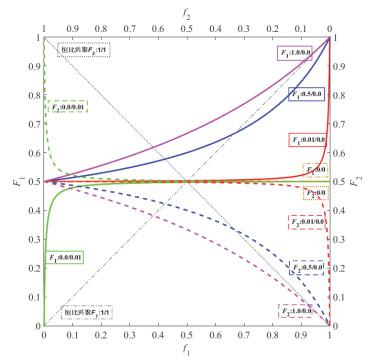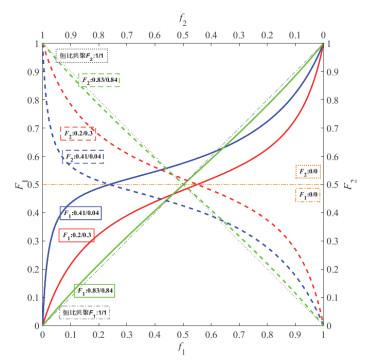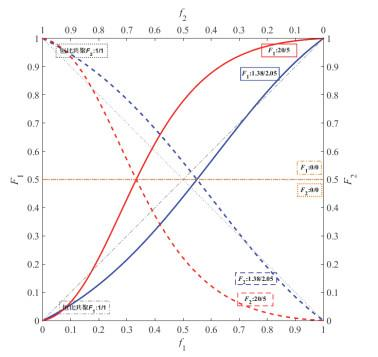## Using the Plot Function in Matlab to Draw the Hyperbolic Curve of Binary Copolymer Composites

Ding Xuyin, Cun Fei, Xie Meiran,

 基金资助: 2017年度上海市教委本科重点课程建设项目2016年度华东师范大学在线教学平台课程建设项目

 Fund supported: 2017年度上海市教委本科重点课程建设项目2016年度华东师范大学在线教学平台课程建设项目Abstract

The Matrix Laboratory (Matlab) software was used to program the Plot function, and the hyperbolic curves of binary copolymer composites were drawn successfully by inputting the reactivity ratio of monomers. From the hyperbolic curve, the binary copolymerization behavior of two monomers can be observed simultaneously, and the varying regularity between the second monomer and the corresponding polymer composition can be visualized in one graph, which will be helpful to deepen the understanding for the copolymerization reaction of two monomers.

Keywords： Matlab ; Plot Function ; Reactivity Ratio ; Binary Copolymer Composite ; Hyperbolic Curve

Ding Xuyin. Using the Plot Function in Matlab to Draw the Hyperbolic Curve of Binary Copolymer Composites. University Chemistry[J], 2020, 35(1): 105-110 doi:10.3866/PKU.DXHX201904007

$\begin{array}{l}{F_1} = {\rm{d}}[{{\rm{M}}_1}\left] {/({\rm{d}}} \right[{{\rm{M}}_1}\left] { + {\rm{d}}} \right[{{\rm{M}}_2}]) = ({r_1}f_1^2 + {f_1}{f_2})/({r_1}f_1^2 + 2{f_1}{f_2} + {r_2}f_2^2),或\\{F_2} = 1 - {F_1} = {\rm{d}}[{{\rm{M}}_2}\left] {/({\rm{d}}} \right[{{\rm{M}}_1}\left] { + {\rm{d}}} \right[{{\rm{M}}_2}\left] {) = 1 - } \right[({r_1}f_1^2 + {f_1}{f_2})/({r_1}f_1^2 + 2{f_1}{f_2} + {r_2}f_2^2)]\end{array}$

F1 (或F2)代表单元M1 (或M2)占共聚物组成的摩尔分数。其中，f1 = 1 - f2 = [M1]/([M1]+ [M2])，f1等于某瞬间单元M1占单体混合物([M1]+ [M2])的摩尔分数。

Matlab是一种高级的计算机语言，具有语言简洁、库函数和运算符丰富、图形功能强大等特点，它不仅可以通过简单的程序语言编写绘制出所需的函数，而且可以对绘制的图形进行各种编辑，如图例、线段种类、坐标轴范围等。但是，文献报道中使用Matlab软件绘制二元共聚物组成曲线，一般都需要具备一定的编程能力[3, 4]，对于不擅长或者不会编程以及不熟悉Matlab软件的学习者来说，需要花费很多的时间和精力学习这些知识之后，才能将二元共聚曲线绘制出来。此外，Origin软件是科研工作者常用的作图软件，利用Origin 8.0中函数绘图的功能，可以编辑共聚物组成方程，进而绘制二元共聚曲线。但是，每次只能针对一个确定的函数进行绘图。如果利用Origin 8.0绘制多条不同竞聚率比的二元共聚双曲线，在绘制每条共聚曲线前都要编辑一个相应共聚物组成的函数，然后通过图层叠加，才能在同一个图中获得一系列的二元共聚双曲线。这种方法不仅耗费大量的时间和精力，而且需要编写多个复杂的函数，出错率也会大大增加。

## 1 Matlab软件编辑Plot函数

 figure ax(1) =gca; set(gcf, 'Position', [30, 30, 700, 720]); set(gca, 'Position', [.115.10.78.8]); f1=0:0.0001:1 for i=1:7 A = [0.1, 0.2, 0.5, 1, 2, 5, 10]; B = [10, 5, 2, 1, 0.5, 0.2, 0.1]; r1 = A(i); r2 = B(i); F1 =(r1*f1.^2+f1-f1.^2)./(r1*f1.^2+2*(f1-f1.^2)+r2*(1-f1).^2); plot(f1, F1, 'linewidth', 2); hold on end xlabel('{\itf}_1'); ylabel('{\itF}_1'); hold on ax(2) = axes('position', get(ax(1), 'position')); f2=1-f1 for i=1:7 A = [0.1, 0.2, 0.5, 1, 2, 5, 10]; B = [10, 5, 2, 1, 0.5, 0.2, 0.1]; r1 = A(i); r2 = B(i); F2=(r2*f2.^2+f2-f2.^2)./(r2*f2.^2+2*(f2-f2.^2)+r1*(1-f2).^2); plot(f2, F2, '--', 'linewidth', 2); hold on end set(ax(1), 'box', 'off'); set(ax(1), 'ylim', [0, 1], 'yTick', [0:0.1:1]); set(ax(2), 'XAxisLocation', 'top', 'YAxisLocation', 'right', 'box', 'off', 'color', 'none', 'XDir', 'reverse'); set(ax(2), 'ylim', [0, 1], 'yTick', [0:0.1:1]); xlabel('{\itf}_2'); ylabel('{\itF}_2');

f1”“f2”“A/a”“B/b”“F1”“F2”分别代表“f1”“f2”“r1”“r2”“F1”“F2

### 图1### 图2r1 < 1, r2 < 1且r1r2→0

### 图3r1r2 < 1且r1 < 1, r2 < 1

### 图4r1r2 < 1且r1 > 1, r2 < 1或r1 < 1, r2 > 1

### 图5r1 > 1且r2 > 1

## 参考文献 原文顺序 文献年度倒序 文中引用次数倒序 被引期刊影响因子

/

 〈〉